Thermodynamics and Propulsion
Subsections

# 2.3 Example Applications of the First Law to motivate the use of a property called enthalpy''

[VW, S & B: 5.4-5.5]

## 2.3.1 Adiabatic, steady, throttling of a gas (flow through a valve or other restriction)

Figure 2.5 shows the configuration of interest. We wish to know the relation between properties upstream of the valve, denoted by 1'' and those downstream, denoted by 2''.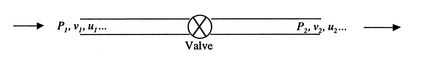To analyze this situation, we can define the system (choosing the appropriate system is often a critical element in effective problem solving) as a unit mass of gas in the following two states. Initially the gas is upstream of the valve and just through the valve. In the final state the gas is downstream of the valve plus just before the valve. The figures on the left of Figure 2.6 show the actual configuration just described. In terms of the system behavior, however, we could replace the fluid external to the system by pistons which exert the same pressure that the external fluid exerts, as indicated schematically on the right side of Figure 2.6.

The process is adiabatic, with changes in potential energy and kinetic energy assumed to be negligible. The first law for the system is therefore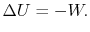The work done by the system is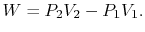Use of the first law leads toIn words, the initial and final states of the system have the same value of the quantity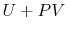. For the case examined, since we are dealing with a unit mass, the initial and final states of the system have the same value of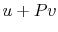.

We define this quantity as the enthalpy,'' usually denoted by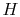,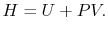In terms of the specific quantities, the enthalpy per unit mass is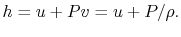It is a function of the state of the system.has units of Joules, and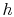has units of Joules per kilogram.

The utility and physical significance of enthalpy will become clearer as we work with more flow problems. For now, you may wish to think of it as follows (Levenspiel, 1996). When you evaluate the energy of an object of volume, you have to remember that the object had to push the surroundings out of the way to make room for itself. With pressureon the object, the work required to make a place for itself is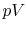. This is so with any object or system, and this work may not be negligible. (The force of one atmosphere pressure on one square meter is equivalent to the force of a mass of about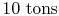.) Thus the total energy of a body is its internal energy plus the extra energy it is credited with by having a volumeat pressure. We call this total energy the enthalpy,.

Muddy Points

When is enthalpy the same in initial and final states? (MP 2.3)

## 2.3.2 Quasi-Static Expansion of a Gas

Consider a quasi-static process of constant pressure expansion. We can write the first law in terms of the states before and after the expansion as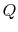and writing the work in terms of system properties,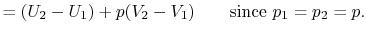By grouping terms we can write the heat input in terms of the enthalpy change of the system: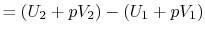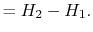## 2.3.3 Transient filling of a tank

Another example of a flow process, this time for an unsteady flow, is the transient process of filling a tank, initially evacuated, from a surrounding atmosphere, which is at a pressure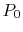and a temperature. The configuration is shown in Figure 2.7.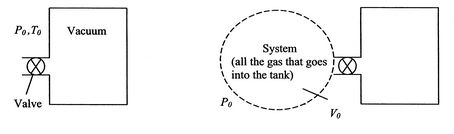At a given time, the valve at the tank inlet is opened and the outside air rushes in. The inflow stops when the pressure inside is equal to the pressure outside. The tank is insulated, so there is no heat transfer to the atmosphere. What is the final temperature of the gas in the tank?

This time we take the system to be all the gas that enters the tank. The initial state has the system completely outside the tank, and the final state has the system completely inside the tank. The kinetic energy initially and in the final state is negligible, as is the change in potential energy, so the first law again takes the formWork is done on the system, of magnitude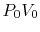, where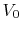is the initial volume of the system, soIn terms of quantities per unit mass (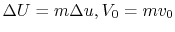, where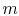is the mass of the system),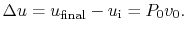The final value of the internal energy is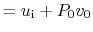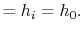For a perfect gas with constant specific heats (see the next section, Section 2.4),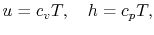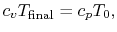The final temperature is thus roughly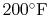hotter than the outside air!

It may be helpful to recap what we used to solve this problem. There were basically four steps:

1. Definition of the system
2. Use of the first law
3. Equating the work to a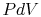'' term
4. Assuming the fluid to be a perfect gas with constant specific heats.

A message that can be taken from both of these examples (as well as from a large number of other more complex situations, is that the quantity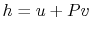occurs naturally in problems of fluid flow. Because the combination appears so frequently, it is not only defined but also tabulated as a function of temperature and pressure for a number of working fluids.

Muddy Points

In the filling of a tank, why (physically) is the final temperature in the tank higher than the initial temperature? (MP 2.4)

## 2.3.4 The First Law in Terms of Enthalpy

We start with the first law in differential form and substitute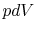for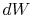by assuming a quasi-static or reversible process: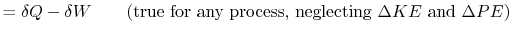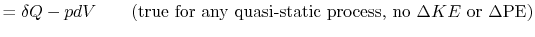The definition of enthalpy,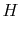can be differentiated (applying the chain rule to theterm) to produce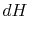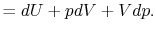Substituting theabove for thein the First Law, we obtain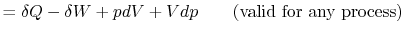or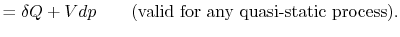Douglas Quattrochi 2006-08-06